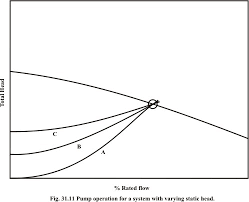## How to Calculate and Solve for Rated Static Head (in Bottom Gating) | Design of Gating SystemThe image above represents rated static head (in bottom gating).

To compute for rated static head, two essential parameters are needed and these parameters are Height of the Sprue from its Top to the Point of Metal Entry into the Mould Cavity (H) and Height of the Casting (C).

The formula for calculating rated static head (in bottom gating).

Hs = H – C / 2

Where:

Hs = Rated Static Head (in Bottom Gating)
H = Height of the Sprue from its Top to the Point of Metal Entry into the Mould Cavity
C = Height of Casting

Let’s solve an example;
Find the rated static head (in bottom gating) when the height of the sprue from its top to the point of metal entry into the mould cavity is 11 and the height of casting is 8.

This implies that;

H = Height of the Sprue from its Top to the Point of Metal Entry into the Mould Cavity = 11
C = Height of Casting = 8

Hs = H – C / 2
Hs = 11 – 8 / 2
Hs = 11 – 4
Hs = 7

Therefore, the rated static head (in bottom gating) is 7 m.

Calculating the Height of the Sprue from its Top to the Point of Metal Entry into the Mould Cavity when the Rated Static Head (in bottom gating) and the Height of Casting is Given.

H = HsC / 2

Where;

H = Height of the Sprue from its Top to the Point of Metal Entry into the Mould Cavity
Hs = Rated Static Head (in Bottom Gating)
C = Height of Casting

Let’s solve an example;
Find the height of the sprue from its top to the point of metal entry into the mould cavity when the rated static head is 22 and the height of casting is 8.

This implies that;

Hs = Rated Static Head (in Bottom Gating) = 22
C = Height of Casting = 8

H = HsC / 2
H = 22 + 8 / 2
H = 22 + 4
H = 26

Therefore, the height of the sprue from its top to the point of metal entry into the mould cavity is 26 m.